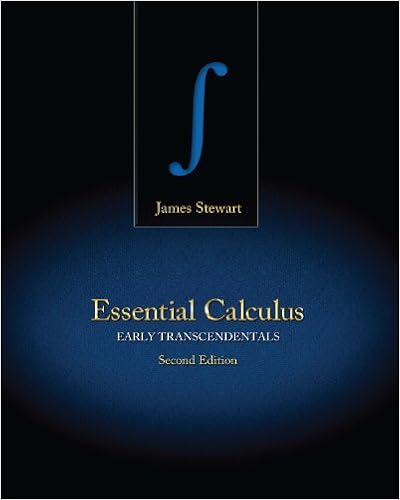# Chapter17_ Second-Order Differential Equations - 17...

• Notes
• mimi304
• 30

This preview shows page 1 - 4 out of 30 pages.

##### We have textbook solutions for you!
The document you are viewing contains questions related to this textbook.The document you are viewing contains questions related to this textbook.
Chapter 7 / Exercise 13
Essential Calculus: Early Transcendentals
StewartExpert Verified
Second-Order Differential Equations 17 The basic ideas of differential equations were explained in Chapter 9; there we concentrated on first-order equations. In this chapter we study second-order linear differential equations and learn how they can be applied to solve problems concerning the vibrations of springs and the analysis of electric circuits. We will also see how infinite series can be used to solve differential equations. 1165 © Christoff / Shutterstock The motion of a shock absorber in a car is described by the differential equations that we solve in Section 17.3.
##### We have textbook solutions for you!
The document you are viewing contains questions related to this textbook.The document you are viewing contains questions related to this textbook.
Chapter 7 / Exercise 13
Essential Calculus: Early Transcendentals
StewartExpert Verified
1166 CHAPTER 17 17.1 Second-Order Linear Equations A second-order linear differential equation has the form where , , , and are continuous functions. We saw in Section 9.1 that equations of this type arise in the study of the motion of a spring. In Section 17.3 we will further pur- sue this application as well as the application to electric circuits. In this section we study the case where , for all , in Equation 1. Such equa- tions are called homogeneous linear equations. Thus the form of a second-order linear homo- geneous differential equation is If for some , Equation 1 is nonhomogeneous and is discussed in Section 17.2. Two basic facts enable us to solve homogeneous linear equations. The first of these says that if we know two solutions and of such an equation, then the linear combination is also a solution. Theorem If and are both solutions of the linear homogeneous equation and and are any constants, then the function is also a solution of Equation 2. PROOF Since and are solutions of Equation 2, we have and Therefore, using the basic rules for differentiation, we have Thus is a solution of Equation 2. P x d 2 y dx 2 Q x dy dx R x y G x G R Q P x G x 0 P x d 2 y dx 2 Q x dy dx R x y 0 x G x 0 y 2 y 1 y c 1 y 1 c 2 y 2 y 2 x y 1 x c 2 c 1 y x c 1 y 1 x c 2 y 2 x y 2 y 1 P x y 1 Q x y 1 R x y 1 0 P x y 2 Q x y 2 R x y 2 0 P x y Q x y R x y P x c 1 y 1 c 2 y 2 Q x c 1 y 1 c 2 y 2 R x c 1 y 1 c 2 y 2 P x c 1 y 1 c 2 y 2 Q x c 1 y 1 c 2 y 2 R x c 1 y 1 c 2 y 2 c 1 P x y 1 Q x y 1 R x y 1 c 2 P x y 2 Q x y 2 R x y 2 c 1 0 c 2 0 0 y c 1 y 1 c 2 y 2 1 2 3 2
SECOND-ORDER LINEAR EQUATIONS 1167 The other fact we need is given by the following theorem, which is proved in more advanced courses. It says that the general solution is a linear combination of two linearly independent solutions and This means that neither nor is a constant multiple of the other. For instance, the functions and are linearly dependent, but and are linearly independent. Theorem If and are linearly independent solutions of Equation 2 on an interval, and is never 0, then the general solution is given by where and are arbitrary constants. Theorem 4 is very useful because it says that if we know two particular linearly inde- pendent solutions, then we know every solution.
•••